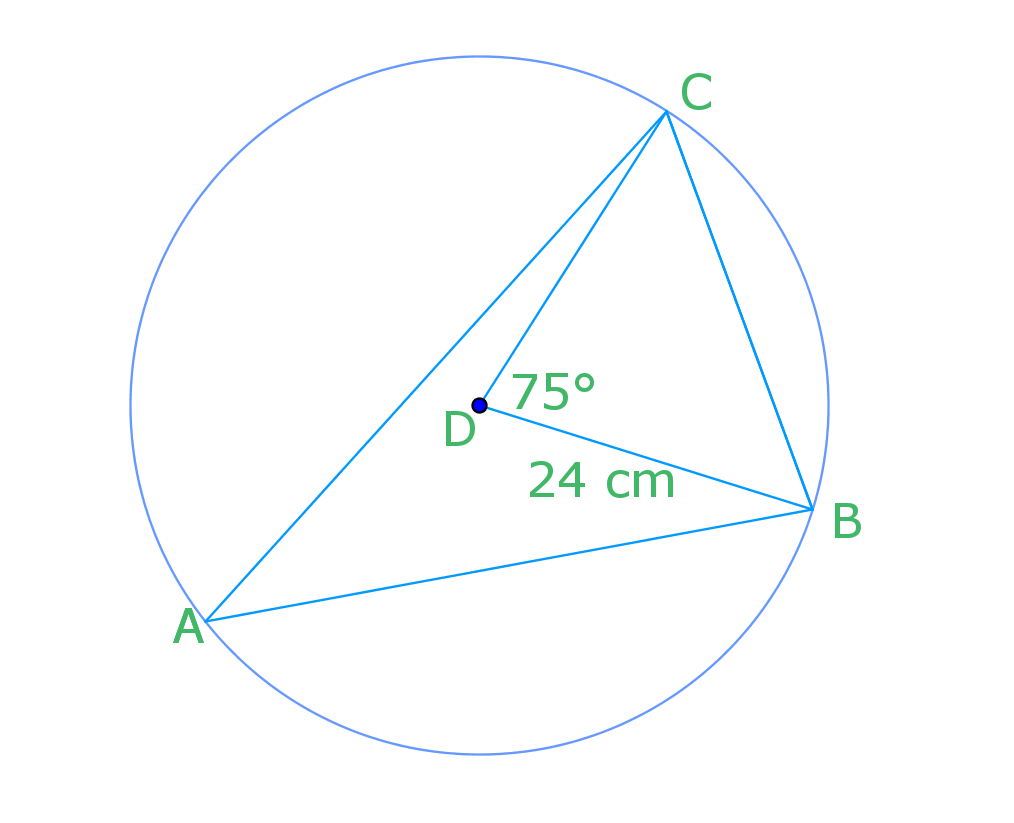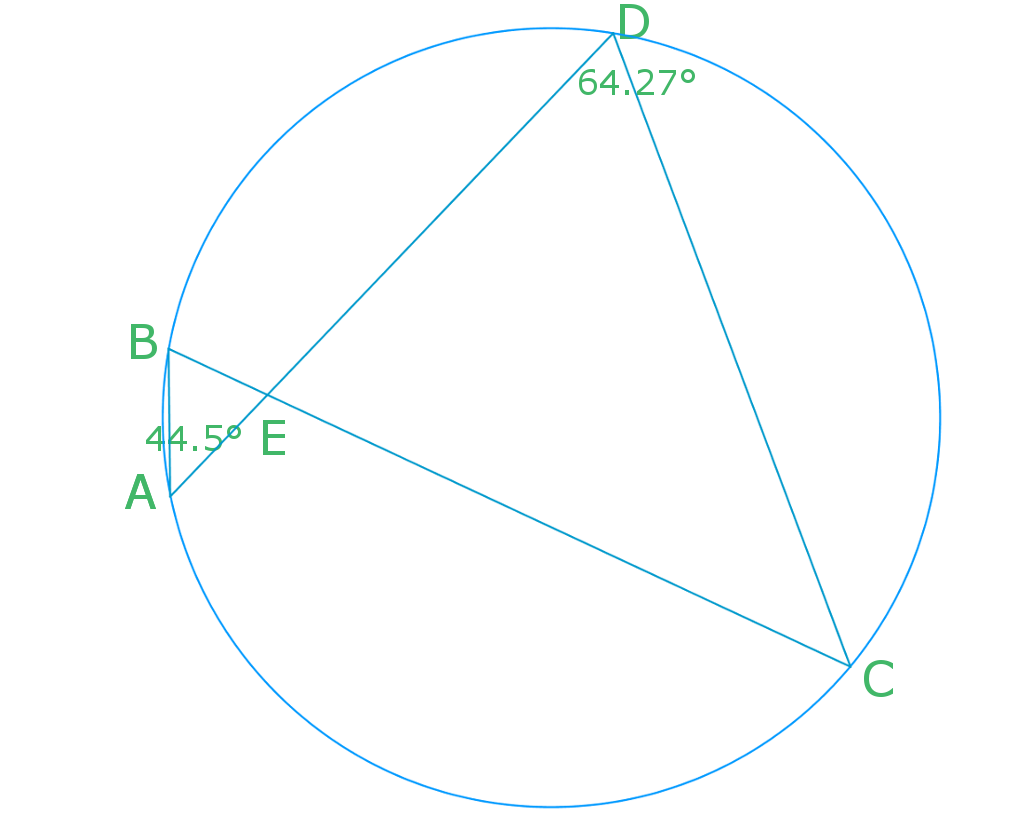# Angles in a circle#### All in One Place

Everything you need for better grades in university, high school and elementary.#### Learn with Ease

Made in Canada with help for all provincial curriculums, so you can study in confidence.#### Instant and Unlimited Help

0/2
##### Intros
###### Lessons
1. Terms related to circles
• Diameter
• Circumference
• Central angle
• minor arc
• major arc
• Inscribed angle
• Chord
• perpendicular bisector
• Tangent
• point of tangency
2. What are Inscribed angles and Central angles?
0/6
##### Examples
###### Lessons
1. In the following diagram, the radius is 24 cm and $\angle$BDC is 75°.1. Find $\angle$BAC.
2. Find the chord BC.
2. You are setting up a display board for the science fair. You have two spotlights: one projects through an angle of 20°; and one projects through an angle of 40°. Where is the best place to put the display board? Show your answer in a diagram.
1. Given $\angle$BAE = 44.5° and $\angle$ADC = 64.27°.Find.
1. $\angle$BCD
2. $\angle$AEB
3. $\angle$CED
###### Topic Notes
In a circle, chords, angles, inscribed angles and arc length all have special relationships with each other. This lesson focuses on exploring the relationships among inscribed angles in a circle as well as those of inscribed angle and central angle with the same arc. We will make use of the relationships to solve related questions in this lesson.

## What is a central angle

Previously, when you worked with angles in a triangle, you were mainly working with central angles. These are angles that had their vertices at the center of the circle. However, in this lesson, we won't be working with central angles.

## What is an inscribed angle

If we're not working with central angles, then what angles in a circle will we be focusing on? We'll be tackling inscribed angles.

Inscribed angles are angles that can be anywhere on the circle's circumference. While we're not solely working with central angles anymore, you'll learn that the central angle of a circle will still come into play when we're going through the example problems in this lesson.

## Inscribed angle theorem

There are several inscribed angle theorems that we'll be learning today. One of them is the angle in the center theorem. This tells us that an inscribed angle $\theta$ is half of the central angle 2?. This is seen illustrated below.

## Angles subtended on the same arc theorem

Another theorem that we'll learn is the angles subtended by the same arc theorem. Given that the endpoints are the same, you'll realize that from the below illustration, it doesn't matter where the inscribed angle $\theta$ is. It will remain the same. As you can also see, this is because they share the same arc (the orange line), hence the name of this theorem.

Question 1:

In the following diagram, the radius is 24 cm and angle BDC is 75°.

a) Find angle BAC.

Solution:

Angle BDC is the central angle, which equals 75 degrees. Angle BAC is the inscribed angle. According to the inscribed angle theorem, angle BAC equals half of angle BDC. Conversely, you can think about the relationship between angle BDC and angle BAC as the central angle always being doubled that of the inscribed angle.

Central angle = inscribed angle x 2

$75$°$=2\theta$

$\theta=37.5$°

b) Find the chord BC

Solution:

Let us draw a bisector in triangle DBC to cut it in half

Now, look at one of the halves cut from triangle DBC

X is half of the chord BC that we are looking for. So solve for x using sin (remember the principles of SohCahToa?)

$BC=2x$

$\sin 37.5$°$=\frac{x}{24}$

$x \cong 14.6$

$BC=2x=29.2cm$

Question 2:

Given angle BAE = 44.5° and angle ADC = 64.27°.